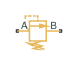# Pressure Relief Valve (TL)

Pressure relief valve in a thermal liquid network

• Library:
• Simscape / Fluids / Thermal Liquid / Valves & Orifices / Pressure Control Valves

•## Description

The Pressure Relief Valve (TL) block represents a valve that relieves excess pressure in a thermal liquid network. The valve allows flow through the networks and opens to relieve excess flow when the control pressure reaches the value of the Valve set pressure (gauge) parameter. A control pressure above the set pressure causes the valve to gradually open, which allows the fluid network to relieve excess pressure.

A smoothing function allows the valve opening area to change smoothly between the fully closed and fully open positions. The smoothing function removes the abrupt opening area changes at the zero and maximum opening positions. The figure shows the effect of smoothing on the valve opening area curve.### Mass Balance

The mass conservation equation in the valve is

`${\stackrel{˙}{m}}_{A}+{\stackrel{˙}{m}}_{B}=0,$`

where:

• ${\stackrel{˙}{m}}_{A}$ is the mass flow rate into the valve through port A.

• ${\stackrel{˙}{m}}_{B}$ is the mass flow rate into the valve through port B.

### Momentum Balance

The momentum conservation equation in the valve is

`${p}_{A}-{p}_{B}=\frac{\stackrel{˙}{m}\sqrt{{\stackrel{˙}{m}}^{2}+{\stackrel{˙}{m}}_{cr}^{2}}}{2{\rho }_{Avg}{C}_{d}^{2}{S}^{2}}\left[1-{\left(\frac{{S}_{R}}{S}\right)}^{2}\right]P{R}_{Loss},$`

where:

• pA and pB are the pressures at port A and port B.

• $\stackrel{˙}{m}$ is the mass flow rate.

• ${\stackrel{˙}{m}}_{cr}$ is the critical mass flow rate.

• ρAvg is the average liquid density.

• Cd is the discharge coefficient.

• SR is the valve opening area.

• S is the valve inlet area.

• PRLoss is the pressure ratio:

`$P{R}_{Loss}=\frac{\sqrt{1-{\left({S}_{R}/S\right)}^{2}\left(1-{C}_{d}^{2}\right)}-{C}_{d}\left({S}_{R}/S\right)}{\sqrt{1-{\left({S}_{R}/S\right)}^{2}\left(1-{C}_{d}^{2}\right)}+{C}_{d}\left({S}_{R}/S\right)}.$`

The block computes the valve opening as

`${S}_{R}={\stackrel{^}{p}}_{smoothed}\cdot \left({S}_{Max}-{S}_{Leak}\right)+{S}_{Leak}$`

`$\stackrel{^}{p}=\frac{{p}_{control}-{p}_{set}}{{p}_{Max}-{p}_{set}}$`

where:

• SLeak is the valve leakage area.

• SMax is the maximum valve opening area.

• pcontrol is the valve control pressure:

• pset is the valve set pressure:

• pMax is the maximum pressure:

where the two equations refer to the settings of the Pressure control specification parameter.

The critical mass flow rate is

`${\stackrel{˙}{m}}_{cr}={\mathrm{Re}}_{cr}{\mu }_{Avg}\sqrt{\frac{\pi }{4}{S}_{R}}.$`

Numerically-Smoothed Valve Area

When the valve is in a near-open or near-closed position, you can maintain numerical robustness in your simulation by adjusting the parameter. If the parameter is nonzero, the block smoothly saturates the control pressure between pset and pMax. For more information, see Numerical Smoothing.

### Energy Balance

The energy conservation equation in the valve is

`${\varphi }_{A}+{\varphi }_{B}=0,$`

where:

• ϕA is the energy flow rate into the valve through port A.

• ϕB is the energy flow rate into the valve through port B.

## Ports

### Conserving

expand all

Thermal liquid conserving port associated with valve inlet A.

Thermal liquid conserving port associated with valve inlet B.

## Parameters

expand all

Specification method for the valve control pressure. When you select `Pressure at port A`, the block only uses the pressure at port A to determine when the flow exceeds the value of the Valve set pressure (gauge) parameter. When you select `Pressure differential`, the block uses the value of the Valve set pressure differential parameter to determine whether the pressure differential from port A to port B exceeds the range.

Pressure at which the opening mechanism triggers. The opening area remains variable throughout the pressure regulation range of the valve.

#### Dependencies

To enable this parameter, set Pressure control specification to ```Pressure pressure at port A```.

Minimum pressure differential between ports A and B required to open the valve. A pressure differential above this value causes the valve to gradually open until it reaches the fully open state.

#### Dependencies

To enable this parameter, set Pressure control specification to ```Pressure differential```.

Pressure interval over which the valve opening area varies. The interval begins at the value of the Valve set pressure (gauge) or once the difference between ports A and B exceeds the value of the Valve set pressure differential parameter.

Opening area of the valve in the fully open position, when the valve is at the upper limit of the pressure regulation range.

Sum of all gaps when the valve is in the fully shut position. The block saturates smaller numbers to this value. This parameter contributes to numerical stability by maintaining continuity in the flow.

Continuous smoothing factor that introduces a layer of gradual change to the flow response when the valve is in near-open or near-closed positions. Set this value to a nonzero value less than one to increase the stability of your simulation in these regimes.

A smoothing factor of 0 corresponds to a linear function that is discontinuous at the set and maximum-area pressures. A smoothing factor of 1 corresponds to a nonlinear function that changes continuously throughout the entire function domain.

A smoothing factor between 0 and 1 corresponds to a continuous function with smooth nonlinear transitions at the set and maximum-area pressures. The figure shows the smoothing regions.Area at ports A and B, which are used in the pressure-flow rate equation that determines the mass flow rate through the valve.

Correction factor that accounts for discharge losses in theoretical flows.

Upper Reynolds number limit for laminar flow through the valve.

## Version History

Introduced in R2016a